Anzeige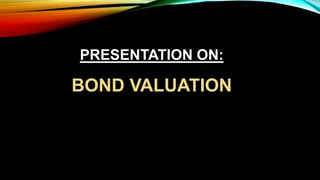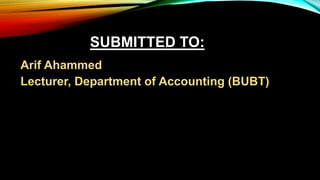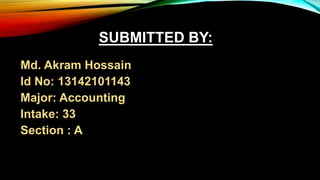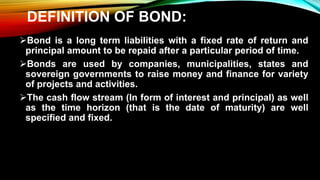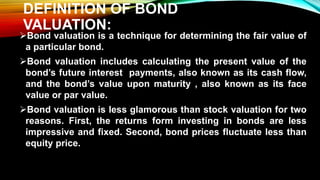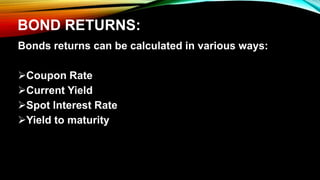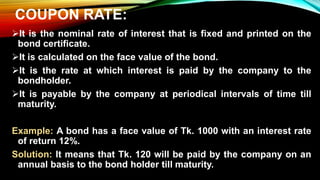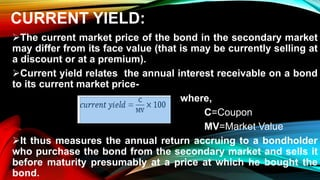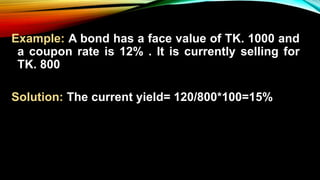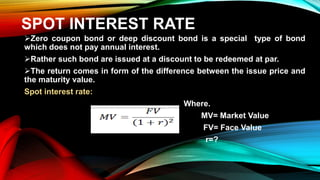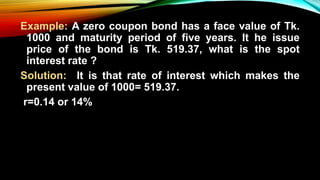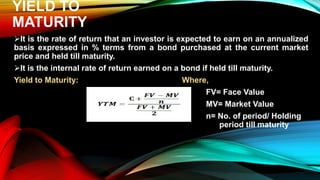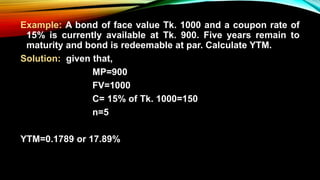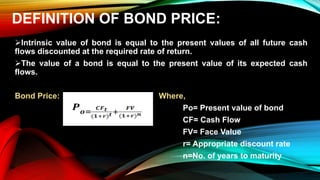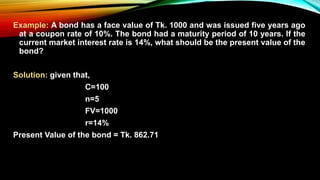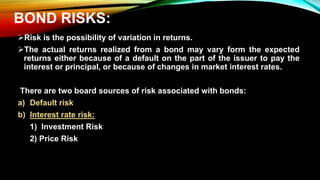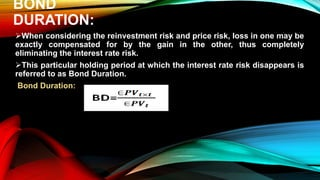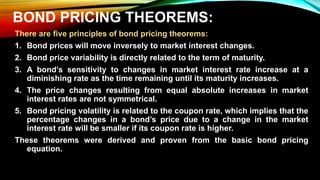1 von 20
Anzeige

### Security Analysis & Portfolio Management

1. WELCOME TO MY PRESENTATION
2. PRESENTATION ON: BOND VALUATION
3. SUBMITTED TO: Arif Ahammed Lecturer, Department of Accounting (BUBT)
4. SUBMITTED BY: Md. Akram Hossain Id No: 13142101143 Major: Accounting Intake: 33 Section : A
5. DEFINITION OF BOND: Bond is a long term liabilities with a fixed rate of return and principal amount to be repaid after a particular period of time. Bonds are used by companies, municipalities, states and sovereign governments to raise money and finance for variety of projects and activities. The cash flow stream (In form of interest and principal) as well as the time horizon (that is the date of maturity) are well specified and fixed.
6. DEFINITION OF BOND VALUATION: Bond valuation is a technique for determining the fair value of a particular bond. Bond valuation includes calculating the present value of the bond’s future interest payments, also known as its cash flow, and the bond’s value upon maturity , also known as its face value or par value. Bond valuation is less glamorous than stock valuation for two reasons. First, the returns form investing in bonds are less impressive and fixed. Second, bond prices fluctuate less than equity price.
7. BOND RETURNS: Bonds returns can be calculated in various ways: Coupon Rate Current Yield Spot Interest Rate Yield to maturity
8. COUPON RATE: It is the nominal rate of interest that is fixed and printed on the bond certificate. It is calculated on the face value of the bond. It is the rate at which interest is paid by the company to the bondholder. It is payable by the company at periodical intervals of time till maturity. Example: A bond has a face value of Tk. 1000 with an interest rate of return 12%. Solution: It means that Tk. 120 will be paid by the company on an annual basis to the bond holder till maturity.
9. CURRENT YIELD: The current market price of the bond in the secondary market may differ from its face value (that is may be currently selling at a discount or at a premium). Current yield relates the annual interest receivable on a bond to its current market price- where, C=Coupon MV=Market Value It thus measures the annual return accruing to a bondholder who purchase the bond from the secondary market and sells it before maturity presumably at a price at which he bought the bond.
10. Example: A bond has a face value of TK. 1000 and a coupon rate is 12% . It is currently selling for TK. 800 Solution: The current yield= 120/800*100=15%
11. SPOT INTEREST RATE Zero coupon bond or deep discount bond is a special type of bond which does not pay annual interest. Rather such bond are issued at a discount to be redeemed at par. The return comes in form of the difference between the issue price and the maturity value. Spot interest rate: Where. MV= Market Value FV= Face Value r=?
12. Example: A zero coupon bond has a face value of Tk. 1000 and maturity period of five years. It he issue price of the bond is Tk. 519.37, what is the spot interest rate ? Solution: It is that rate of interest which makes the present value of 1000= 519.37. r=0.14 or 14%
13. YIELD TO MATURITY It is the rate of return that an investor is expected to earn on an annualized basis expressed in % terms from a bond purchased at the current market price and held till maturity. It is the internal rate of return earned on a bond if held till maturity. Yield to Maturity: Where, FV= Face Value MV= Market Value n= No. of period/ Holding period till maturity
14. Example: A bond of face value Tk. 1000 and a coupon rate of 15% is currently available at Tk. 900. Five years remain to maturity and bond is redeemable at par. Calculate YTM. Solution: given that, MP=900 FV=1000 C= 15% of Tk. 1000=150 n=5 YTM=0.1789 or 17.89%
15. DEFINITION OF BOND PRICE: Intrinsic value of bond is equal to the present values of all future cash flows discounted at the required rate of return. The value of a bond is equal to the present value of its expected cash flows. Bond Price: Where, Po= Present value of bond CF= Cash Flow FV= Face Value r= Appropriate discount rate n=No. of years to maturity
16. Example: A bond has a face value of Tk. 1000 and was issued five years ago at a coupon rate of 10%. The bond had a maturity period of 10 years. If the current market interest rate is 14%, what should be the present value of the bond? Solution: given that, C=100 n=5 FV=1000 r=14% Present Value of the bond = Tk. 862.71
17. BOND RISKS: Risk is the possibility of variation in returns. The actual returns realized from a bond may vary form the expected returns either because of a default on the part of the issuer to pay the interest or principal, or because of changes in market interest rates. There are two board sources of risk associated with bonds: a) Default risk b) Interest rate risk: 1) Investment Risk 2) Price Risk
18. BOND DURATION: When considering the reinvestment risk and price risk, loss in one may be exactly compensated for by the gain in the other, thus completely eliminating the interest rate risk. This particular holding period at which the interest rate risk disappears is referred to as Bond Duration. Bond Duration:
19. BOND PRICING THEOREMS: There are five principles of bond pricing theorems: 1. Bond prices will move inversely to market interest changes. 2. Bond price variability is directly related to the term of maturity. 3. A bond’s sensitivity to changes in market interest rate increase at a diminishing rate as the time remaining until its maturity increases. 4. The price changes resulting from equal absolute increases in market interest rates are not symmetrical. 5. Bond pricing volatility is related to the coupon rate, which implies that the percentage changes in a bond’s price due to a change in the market interest rate will be smaller if its coupon rate is higher. These theorems were derived and proven from the basic bond pricing equation.
Anzeige